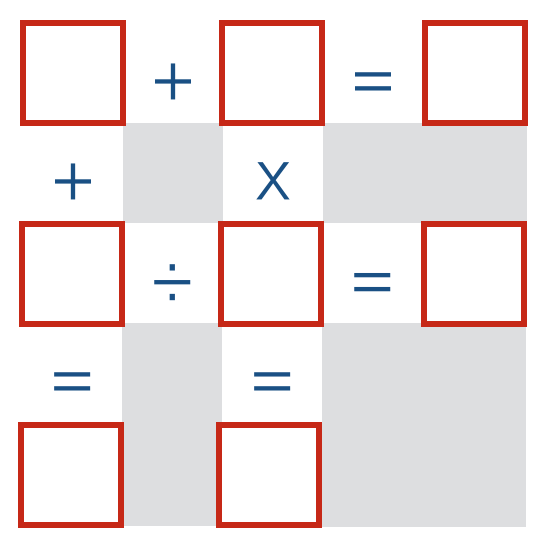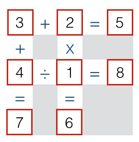# Number Grid Puzzle

Logic Level 1Place each of the digits $1,2,3,\ldots,8$ in the grid above so that all of the equations are true.

Give your answer as the numbers from left-to-right and then top-to-bottom. For example, if you filled in the grid (incorrectly) as shown below, your answer would be $32541876.$×